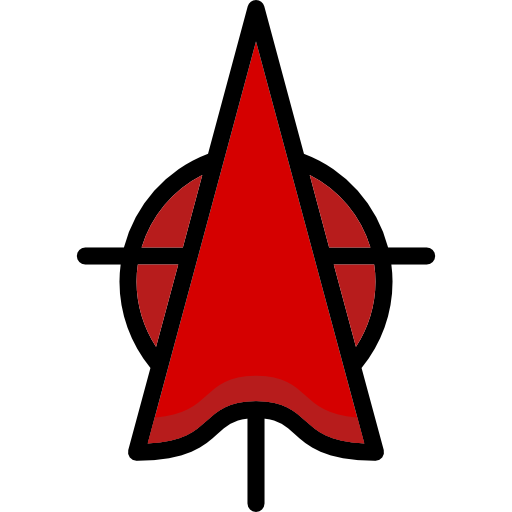# Download PNG image - Line Triangle Red Free PNG HQ#### Share:

Line Triangle Red Free PNG HQ has a transparent background.
This PNG has a resolution of 512x512. You can download the PNG for free in the best resolution and use it for design and other purposes. Line Triangle Red Free PNG HQ just click on Download and save.

A triangle is one of the most basic shapes in geometry. It is a polygon with 3 vertices and 3 edges. There are 7 types of triangles- isosceles (any 2 sides equal), scalene (no side equal), equilateral (all sides equal), right angled ( one angle 90°), acute angled (all angles less than 90°), obtuse angled (one angle greater than 90°) and equiangular (all interior angles are equal). The study of triangle is called the triangle geometry. A triangle is said to be valid if the sum of two sides is greater than that of third side. Because of its unique shape, it is considered very ideal for both architects and students.
These are very commonly found and structurally sound for use in daily life. The strength of this shape spreads force equally between its three sides. Triangles are used for construction purposes. They are used to make rafters in buildings and curved domes. Egyptians made triangular shaped pyramids as well. Triangulation is also very much used to calculate the distance of a point from two other points of known distance apart. They have applicability in astronomy, architecture, engineering, physics, navigation and surveying. On joining more number of triangles together, we get different shapes such as hexagon, octagon and so on.

Image Name: Line Triangle Red Free PNG HQ
Image category: Triangle | Download Triangle Zip
Parent category: Animals
Format: PNG image with alpha (transparent)
Resolution: 512x512
License: Creative Commons (CC BY-NC 4.0)
Size: 18.0KB
Rating: 5
Views: 446
Author: Hannah Hill